Integration By Partial Fractions
Integration By Partial Fractions

Integral of ∫4/(x^3 + 4x)dx – do we need partial fractions?

When initially looking at the integral of 4/(x3+4x), your first inclination might be “this is a partial fractions problem” and solve the integral accordingly. So with…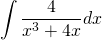You might rewrite the integrand as follows……and solve for the coefficients A, B and C. There is certainly nothing wrong with this approach, but it requires a little more work than the one that we’re about to do.

As mathematicians, we are lazy and we always look for the path of least resistance when it comes to solving problems. So here’s the lazier ways to approach this problem…

Let’s call the integrand p(x). If we can write the integrand in the form of…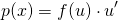…(i.e. in “chain rule” form) then we can use a u-substitution. Better yet, if we can write the integrand in the form of……then we can simply use the standard integral: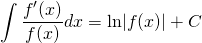So how to we to this with 4/(x3+4x)? Well, let’s factor out an x3 from the denominator term. So…Thus, integrating both sides, we get…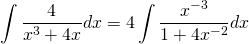Now, we can make the substitution…Therefore, the integral becomes…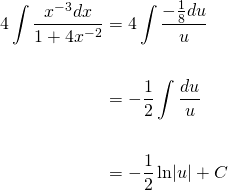Completing by substituting 1+4x-2 back in for u…Ok great! That’s the correct answer. But what about the f'(x)/f(x) approach? Well, with…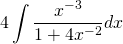The numerator’s power is already of the correct order for the derivative of the denominator. We simply need to multiply it by -8 to make it the derivative. But if we multiply by -8, we also need to multiply by its reciprocal, -1/8 to cancel out its effect. Thus…Therefore…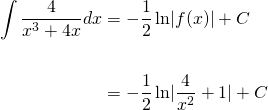Please give me an Upvote and Resteem if you have found this tutorial helpful.